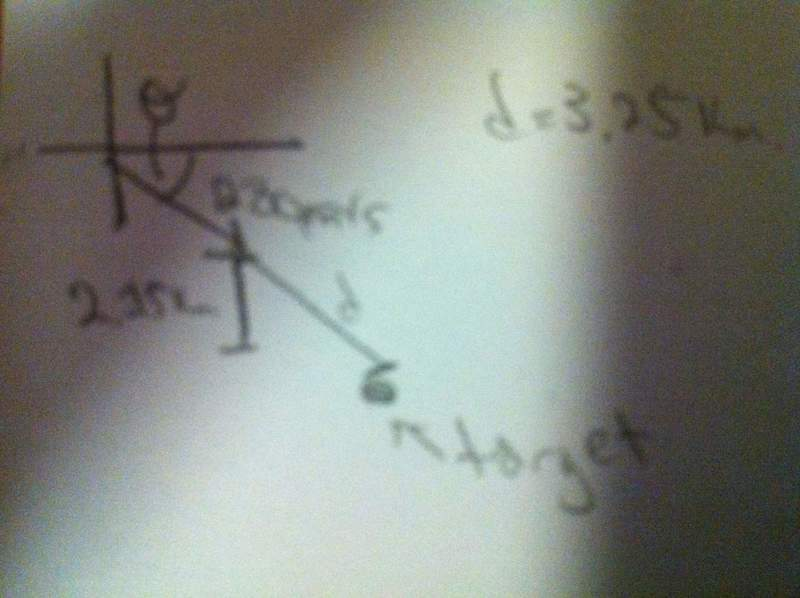# A plane releases a water bomb after reaching a certain altitude. Find Angle

1. A plane has a velocity of 280 m/s at an angle θ below the horizontal. When the altitude of the aircraft is 2.15km, it releases a water bomb, which subsequently hits a target on the ground. The magnitude of the displacement from the point of release of the bomb to the target is 3.25km. Find angle θ

Please help! I've tried drawing the diagram and even that is ending up confusing. I'm quite sure the second triangle coming off the airplane has a y component of 2.15km, and a hypotenuse of 3.25km but I can't determine how the θ of the plane from the horizontal relates to this second triangle.

Last edited by a moderator:

2022 Award
A plane capable of doing a substantial fraction of the speed of sound being used for water bombing? Hm.

From your description of your diagram, I think you are assuming that the bomb travels in a straight line after being released. This isn't the case - it curves towards the ground. You've been given initial velocities in the horizontal and vertical directions (in terms of θ) and distances traveled in the horizontal and vertical directions after some time. Can you relate these pieces of information?

szimmy
A plane capable of doing a substantial fraction of the speed of sound being used for water bombing? Hm.

From your description of your diagram, I think you are assuming that the bomb travels in a straight line after being released. This isn't the case - it curves towards the ground. You've been given initial velocities in the horizontal and vertical directions (in terms of θ) and distances traveled in the horizontal and vertical directions after some time. Can you relate these pieces of information?

I think the problem is stating that if you would draw a direct line from where the bomb was dropped to where it landed it would have traveled 3.25km, not saying the total distance the bomb traveled. From the way he is describing it it seems like it would be possible to solve it by using trigonometry, like a vector problem

I think the problem is stating that if you would draw a direct line from where the bomb was dropped to where it landed it would have traveled 3.25km, not saying the total distance the bomb traveled. From the way he is describing it it seems like it would be possible to solve it by using trigonometry, like a vector problem

Yeah that's what I'm trying to do, but I just can't seem to figure it out.

szimmy
Yeah that's what I'm trying to do, but I just can't seem to figure it out.

Just set up your triangle, with the hypotenuse and the y component, then depending on how you draw it use either arcsin(if you made y opposite theta) or acrcos (if you made it adjacent) to solve for theta

I'm trying to but I can't figure out where to put theta in my new triangle. I'll insert a pic of what I THINK it looks like:It's kind of blurry but it wouldn't focus, sorry. Hopefully you get the gist of it

2022 Award
I read the problem as saying that 3.25km is the horizontal distance, not the hypotenuse. In any case, the point is that the bomb does not follow the hypotenuse to the target. In your diagram, the pilot has pointed the nose of the plane at the target and released the bomb. If he doesn't change course, he will fly into the target and the bomb, dropping downwards under gravity, will drop short.

In other words, theta is not the angle you appear to think that it is. You need to think about the motion of the bomb, not the plane.

Perhaps a simpler question: if the plane were traveling horizontally at 280m/s at 2.15km altitude and released its bomb, how far along the ground would the bomb travel?

I figured it out. It ends up as projectile motion with an initial velocity, thanks :)

2022 Award
That's it - well done.

McDonell
I figured it out. It ends up as projectile motion with an initial velocity, thanks :)

I am working on the same problem. When you do the problem, did you have to solve for time in order to be able to move forward in the question?

Yes but you'll get an expression with theta in it, not a numerical value

McDonell
What equation did you use to start?

V=d/t

McDonell
I solved the question for myself, but I am wondering if I have done it properly. Mind if I ask what your answer was? I got 48 degrees

azizlwl
Knowns=x, y , u and g.
Unknowns=θ

Taking downward as positive.
$y=xTan\theta+\frac{gx^2}{2u^2Cos^2\theta}$

Cos2θ=1-Sin2θ
1/Cos2=1+tan2θ

Last edited:
McDonell
Knowns=x, y , u and g.
Unknowns=θ

Taking downward as positive.
$y=xTan\theta+\frac{gx^2}{2u^2Cos^2\theta}$

Cos2θ=1-Sin2θ
1/Cos2=1+tan2θ

azizlwl
y=vyt + 1/2 at2 ...(1)
x=vxt => t=x/vx...(2)

Sub (2) to (1)

y=vy(x/vx) + 1/2 a(x/vx)2
y=xTanθ+1/2 a(x/vCosθ)2

McDonell
Ok I understand how you formulated this equation. Now I can plug my numbers in and I get

y=xTanθ+1/2 a(x/vCosθ)2

2150 = 3250Tanθ + 9.8(3250)2) / 2(280)2cos2θ

How do I get rid of the tan and cos?

azizlwl
Ok I understand how you formulated this equation. Now I can plug my numbers in and I get

y=xTanθ+1/2 a(x/vCosθ)2

2150 = 3250Tanθ + 9.8(3250)2) / 2(280)2cos2θ

How do I get rid of the tan and cos?

Knowns=x, y , u and g.
Unknowns=θ

Taking downward as positive.
$y=xTan\theta+\frac{gx^2}{2u^2Cos^2\theta}$

Cos2θ=1-Sin2θ
1/Cos2=1+tan2θ

Just subtitute 1/Cosθ2 with 1+tan2θ then you have a quadratic equation.

Azizlwl's got it right, solve that using trig identities he stated below.

As for my answer, I got θ=23°

McDonell
Just subtitute 1/Cosθ2 with 1+tan2θ then you have a quadratic equation.

Azizlwl's got it right, solve that using trig identities he stated below.

As for my answer, I got θ=23°